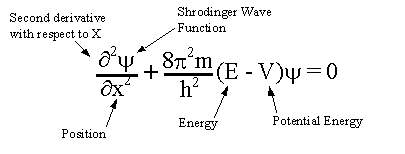QUESTION #329

# What is the Schrodinger equation, and how is it used?

At the beginning of the twentieth century, experimental evidence suggested that atomic particles were also wave-like in nature. For example, electrons were found to give diffraction patterns when passed through a double slit in a similar way to light waves. Therefore, it was reasonable to assume that a wave equation could explain the behaviour of atomic particles.

Schrodinger was the first person to write down such a wave equation. Much discussion then centred on what the equation meant. The eigenvalues of the wave equation were shown to be equal to the energy levels of the quantum mechanical system, and the best test of the equation was when it was used to solve for the energy levels of the Hydrogen atom, and the energy levels were found to be in accord with Rydberg's Law.

It was initially much less obvious what the wavefunction of the equation was. After much debate, the wavefunction is now accepted to be a probability distribution. The Schrodinger equation is used to find the allowed energy levels of quantum mechanical systems (such as atoms, or transistors). The associated wavefunction gives the probability of finding the particle at a certain position.
Answered by: Ian Taylor, Ph.D., Theoretical Physics (Cambridge), PhD (Durham), UK

The Shrodinger equation is:The solution to this equation is a wave that describes the quantum aspects of a system. However, physically interpreting the wave is one of the main philosophical problems of quantum mechanics.

The solution to the equation is based on the method of Eigen Values devised by Fourier. This is where any mathematical function is expressed as the sum of an infinite series of other periodic functions. The trick is to find the correct functions that have the right amplitudes so that when added together by superposition they give the desired solution.

So, the solution to Schrondinger's equation, the wave function for the system, was replaced by the wave functions of the individual series, natural harmonics of each other, an infinite series. Shrodinger has discovered that the replacement waves described the individual states of the quantum system and their amplitudes gave the relative importance of that state to the whole system.

Schrodinger's equation shows all of the wave like properties of matter and was one of greatest achievements of 20th century science.

It is used in physics and most of chemistry to deal with problems about the atomic structure of matter. It is an extremely powerful mathematical tool and the whole basis of wave mechanics.
Answered by: Simon Hooks, Physics A-Level Student, Gosport, UK

The Schrodinger equation is the name of the basic non-relativistic wave equation used in one version of quantum mechanics to describe the behaviour of a particle in a field of force. There is the time dependant equation used for describing progressive waves, applicable to the motion of free particles. And the time independent form of this equation used for describing standing waves.

Schrodinger's time-independent equation can be solved analytically for a number of simple systems. The time-dependant equation is of the first order in time but of the second order with respect to the co-ordinates, hence it is not consistent with relativity. The solutions for bound systems give three quantum numbers, corresponding to three co-ordinates, and an approximate relativistic correction is possible by including fourth spin quantum number.